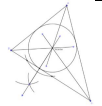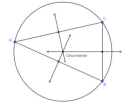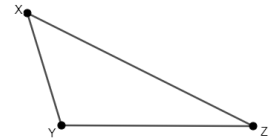# MA.912.GR.5.3Export Print
Construct the inscribed and circumscribed circles of a triangle.

### Clarifications

Clarification 1: Instruction includes using compass and straightedge, string, reflective devices, paper folding or dynamic geometric software.
General Information
Subject Area: Mathematics (B.E.S.T.)
Strand: Geometric Reasoning
Status: State Board Approved

## Benchmark Instructional Guide

### Terms from the K-12 Glossary

• Inscribed Circle
• Circumscribed Circle
• Triangle

### Vertical Alignment

Previous Benchmarks

Next Benchmarks

### Purpose and Instructional Strategies

In grade 7, students used a relationship between triangles and circles to understand the formula for the area of a circle. In Geometry, students identify and construct two special circles that are associated with a triangle.
• Instruction includes the use of manipulatives, tools and geometric software. Allowing students to explore constructions with dynamic software reinforces why the constructions work.
• Instruction includes the student understanding that in a geometric construction, one does not use the markings on a ruler or on a protractor to construct inscribed and circumscribed circles of a triangle. Students should realize that there are limitations on precision that are inherent in the markings on rulers or protractors.
• It is important to build the understanding that formal constructions are valid when the lengths of segments or measures of angles are not known, or have values that do not appear on a ruler or protractor, including irrational values.
• Instruction includes the connection to logical reasoning and visual proofs when verifying that a construction works.
• Instruction includes the connection to constructing angle bisectors and perpendicular bisectors. (MTR.2.1)
• Instruction includes using various methods, like the one described below, to construct an inscribed circle.
• For example, given triangle ABC, students can construct two of the three angle bisectors to create their point of intersection, D. Students should realize that the point D is the incenter of the triangle and may predict that point D will be the center of the inscribed circle. To prove this prediction, students will need to prove that point D is equidistant from each of the three sides. In order to prove this, students can construct the perpendicular segments from point D to each of the three sides, and show that all three segments are congruent using triangle congruence criteria and D is the intersection of the angle bisectors. Each of these segments will be a radius of the inscribed circle, with the center of the circle at point D• When constructing an inscribed circle, students should make the connection to constructing perpendicular bisectors when they need to construct a line through the incenter of the triangle that is perpendicular to a side of the triangle.
• For example, to construct such a line, students can place the compass at the incenter, point D, and draw arcs to determine two points, E and F, on one of the sides. These points are equidistant to D. Then they using the same compass setting, place the compass at E and at F and draw arcs intersecting on the opposite side of EF from D. The intersection of these arcs, Q, is the same distance to E and to F. Therefore, the line passing thru D and Q is the perpendicular bisector of EF so it is also a line perpendicular to the side of the triangle.
• Students should understand that the shortest segment from a point, D, to a line is the segment from D to the line that is perpendicular to the line. Additionally, students should understand that the circle centered at point D, which has this segment as a radius, is tangent to the line.
• Instruction includes using various methods, like the one described below, to construct a circumscribed circle.
• For example, given triangle ABC, students can construct two of the three perpendicular bisectors of the sides of the triangle to create their point of intersection, D. Students should realize that the point D is the circumcenter of the triangle and may predict that point D will be the center of the circumscribed circle. To prove this prediction, students will need to prove that point D is equidistant from each of the three vertices. In order to prove this, students can use the fact that point D is the intersection of the perpendicular bisectors. Each of these segments will be a radius of the circumscribed circle, with the center of the circle at point D. So, to construct the circumscribed circle, one can set the compass equal to the distance between point D and any one of the vertices and then draw the circle centered at point D• Instruction includes exploring the construction of circumscribed circles about various triangles. Have students explore acute, right and obtuse triangles, and compare the locations of the circumcenter of each. Students should understand that with a right triangle, the circumcenter is located at the midpoint of the hypotenuse.
• For expectations of this benchmark, constructions should be reasonably accurate and the emphasis is to make connections between the construction steps and the definitions, properties and theorems supporting them.
• While going over the steps of geometric constructions, ensure that students develop vocabulary to describe the steps precisely. (MTR.4.1)
• Problem types include identifying the next step of a construction, a missing step in a construction or the order of the steps in a construction.

### Common Misconceptions or Errors

• Students may think that the when constructing a circumscribed circle, the center of the circle cannot be outside the triangle.

• Part A. Construct angle bisectors for the three interior angles of a triangle using folding paper, a compass and straightedge and geometric software. What do you notice about each method of construction?
• Part B. Repeat Part A with a triangle that is obtuse, isosceles, acute and right. Describe your findings.
• Part C. Using the incenter as the center, a circle can be constructed inscribed in the triangle. How can you determine the radius of that circle, the inscribed circle?
• Part D. Construct the inscribed circle of one of the triangles from Part B.

• Part A. Construct perpendicular bisector for the three sides of a triangle using folding paper, a compass and straightedge and geometric software. What do you notice about each method of construction?
• Part B. Repeat Part A with a triangle that is obtuse, isosceles, acute and right. Describe your findings.
• Part C. Using the circumcenter as the center, a circle can be constructed circumscribed about the triangle. How can you determine the radius of that circle, the circumscribed circle?
• Part D. Construct the circumscribed circle of one of the triangles from Part B.

• Part A. Given the line $l$ and the point P external to the line $l$, construct a perpendicular line, $m$, through point P
• Part B. Use the construction from Part A to construct a line, $n$, that is parallel to the line $l$ and contains the point P.

### Instructional Items

Instructional Item 1
• Construct the circle that is circumscribed about ΔXYZ.*The strategies, tasks and items included in the B1G-M are examples and should not be considered comprehensive.

## Related Courses

This benchmark is part of these courses.
1200400: Foundational Skills in Mathematics 9-12 (Specifically in versions: 2014 - 2015, 2015 - 2022, 2022 and beyond (current))
1206310: Geometry (Specifically in versions: 2014 - 2015, 2015 - 2022, 2022 and beyond (current))
1206320: Geometry Honors (Specifically in versions: 2014 - 2015, 2015 - 2022, 2022 and beyond (current))
1206315: Geometry for Credit Recovery (Specifically in versions: 2014 - 2015, 2015 - 2022, 2022 and beyond (current))
7912065: Access Geometry (Specifically in versions: 2015 - 2022, 2022 and beyond (current))

## Related Access Points

Alternate version of this benchmark for students with significant cognitive disabilities.
MA.912.GR.5.AP.3: Select the inscribed and circumscribed circles of a triangle.

## Related Resources

Vetted resources educators can use to teach the concepts and skills in this benchmark.

## Formative Assessments

The Sprinters’ Race:

Students are given a grid with three points (vertices of a right triangle) representing the starting locations of three sprinters in a race and are asked to determine the center of the finish circle, which is equidistant from each sprinter.

Type: Formative Assessment

Inscribed Circle Construction:

Students are asked to use a compass and straightedge to construct an inscribed circle of an acute scalene triangle.

Type: Formative Assessment

Circumscribed Circle Construction:

Students are asked to use a compass and straightedge to construct a circumscribed circle of an acute scalene triangle.

Type: Formative Assessment

## Lesson Plans

What's the Point? Part 2:

In this lesson, students use a paper-folding technique to discover the properties of angle bisectors. At the conclusion of the activity, students will be able to compare/contrast the points of concurrency of perpendicular and angle bisectors.

Type: Lesson Plan

Circumnavigating the Circumcenter:

Students use the concurrent point of perpendicular bisectors of triangle sides to determine the circumcenter of three points. Students will reason that the circumcenter of the vertices of a polygon is the optimal location for placement of a facility to service all of the needs of sites at the vertices forming the polygon.

Type: Lesson Plan

What's the Point? Part 1:

This is a patty paper-folding activity where students measure and discover the properties of the point of concurrency of the perpendicular bisectors of the sides of a triangle.

Type: Lesson Plan

## Original Student Tutorials

Pennant Company Challenge: Inscribed Circles of Triangles:

Discover how easy it is for Katie to construct an inscribed circular logo on her company's triangular pennant template. If she completes the task first, she will win a \$1000 bonus! Follow along with this interactive tutorial.

Type: Original Student Tutorial

Good as New:

Learn the steps to circumscribe a circle around a triangle in this interactive tutorial about constructions. Grab a compass, straightedge, pencil and paper to follow along!

Type: Original Student Tutorial

## MFAS Formative Assessments

Circumscribed Circle Construction:

Students are asked to use a compass and straightedge to construct a circumscribed circle of an acute scalene triangle.

Inscribed Circle Construction:

Students are asked to use a compass and straightedge to construct an inscribed circle of an acute scalene triangle.

The Sprinters’ Race:

Students are given a grid with three points (vertices of a right triangle) representing the starting locations of three sprinters in a race and are asked to determine the center of the finish circle, which is equidistant from each sprinter.

## Original Student Tutorials Mathematics - Grades 9-12

Good as New:

Learn the steps to circumscribe a circle around a triangle in this interactive tutorial about constructions. Grab a compass, straightedge, pencil and paper to follow along!

Pennant Company Challenge: Inscribed Circles of Triangles:

Discover how easy it is for Katie to construct an inscribed circular logo on her company's triangular pennant template. If she completes the task first, she will win a \$1000 bonus! Follow along with this interactive tutorial.

## Student Resources

Vetted resources students can use to learn the concepts and skills in this benchmark.

## Original Student Tutorials

Pennant Company Challenge: Inscribed Circles of Triangles:

Discover how easy it is for Katie to construct an inscribed circular logo on her company's triangular pennant template. If she completes the task first, she will win a \$1000 bonus! Follow along with this interactive tutorial.

Type: Original Student Tutorial

Good as New:

Learn the steps to circumscribe a circle around a triangle in this interactive tutorial about constructions. Grab a compass, straightedge, pencil and paper to follow along!

Type: Original Student Tutorial

## Parent Resources

Vetted resources caregivers can use to help students learn the concepts and skills in this benchmark.